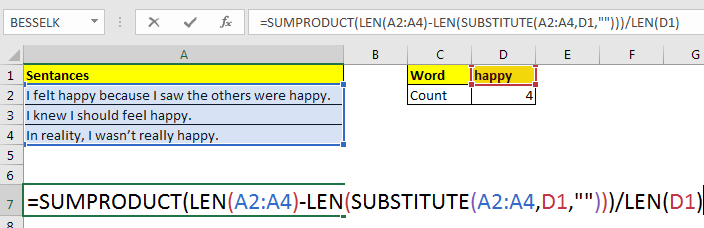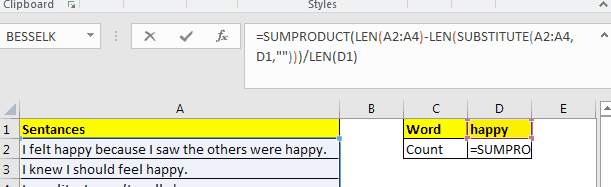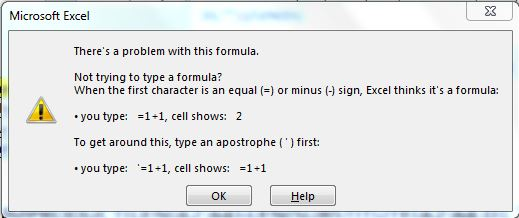# F9 Key to Debug Excel Formulas

To solve formula errors or to evaluate how a complex formula in excel working, one must know how to debug/evaluate excel formulas. In this article, we will learn how to debug or evaluate an excel formula.

## The F9 Excel Formula Checker

The f9 key is used to evaluate the selected segments of the formula and see what they return. You select the part of the formula and press the F9 key to see what that segment of formula returning. Let's see the F9 key in action:

In an article, How to Count Specific Word in an Excel Range we used a "simple" formula:

 =SUMPRODUCT(LEN(range)-LEN(SUBSTITUTE(range,word,"")))/LEN(word)Let's use the F9 key to see which part works how.

For now, I select the A2:A4 and press the F9 key. If you do the same, you will see an array that contains the values of excel range A2:A4. When you select LEN(A2:A4) and press F9 key it will show an array that contains the length of each string in each cell of the range A2:A4 and so on. Whichever part of the formula you will select, the F9 key will show what that part means to that formula.If you select a part that is not a valid expression in for excel formula, and press F9 excel will tell you that the formula is wrong.After using F9 key, if you hit Enter key, the formula will be hardcoded by the values returned by those segments of the formula.

If you just want to check the formula, hit the escape button. If you are an excel formula checker, you would probably use Esc key.

Use of F9 key

Find how the formula works: Sometimes we find excel formulas online and we do get them. We use it and it works. But we are unable to know how it is working. Here F9 key can help you decode those formulas.

Excel Errors: It happens a lot that we write a formula correctly but we get errors in return. In that case, we can use the F9 formula debugger, to see which part of the formula is causing the error. When it comes to solving specific types of errors in excel, we got it covered too.

Logical Errors: These are the hardest type of errors. They don't show any error. They just return an incorrect output and you need to debug why the formula is returning incorrect output. Here F9 key can help you evaluate each segment of the formula to see if it returns the expected output or not. Whichever part does not return the expected value, contains the error.

So yeah guys, this how you can use the F9 key to debug the worksheet formulas. This helps me a lot when I explain how a complex formula is working. I hope it will help you too.

If you have any doubts regarding this article or any other excel/VBA related topic, ask in the comment section below. We will appreciate it and try to resolve the excel problem.

Related Articles:

How to Trace and Fix Formula Errors in Excel | The when a formula that refers to multiple cells and ranges in excel sheet, it gets hard to locate the error. The option Trace Error in Excel helps a lot.

Evaluation of Excel Formula Step By Step | The Evaluate Formula Method is useful when you want to debug your formula from scratch. The Evaluate Formula option shows each steps in which your formula is getting solved. It helps you..

Formula Errors in Excel and Solutions | There are many types of errors that occur in excel. It is good to know which error occurs when in excel. Each error indicates the reason of the error and they have their specific solution in Excel.

Popular Articles:

50 Excel Shortcuts to Increase Your Productivity | Get faster at your task. These 50 shortcuts will make your work even faster on Excel.

The VLOOKUP Function in Excel | This is one of the most used and popular functions of excel that is used to lookup value from different ranges and sheets.

COUNTIF in Excel 2016 | Count values with conditions using this amazing function. You don't need filter your data to count specific value. Countif function is essential to prepare your dashboard.

How to Use the SUMIF Function in Excel | This is another dashboard essential function. This helps you sum up values on specific conditions.

Terms and Conditions of use

The applications/code on this site are distributed as is and without warranties or liability. In no event shall the owner of the copyrights, or the authors of the applications/code be liable for any loss of profit, any problems or any damage resulting from the use or evaluation of the applications/code.